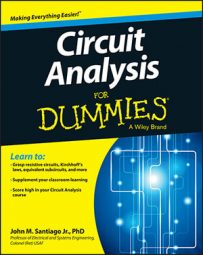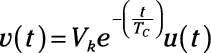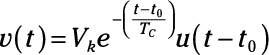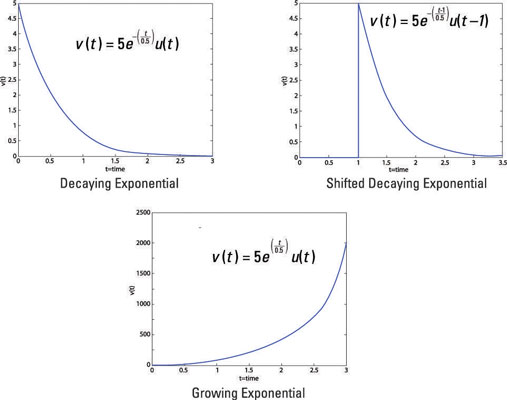##### Circuit Analysis For DummiesThe exponential function is a step function whose amplitude Vk gradually decreases to 0. Exponential functions are important to circuit analysis because they’re solutions to many problems in which a circuit contains resistors, capacitors, and inductors.

The exponential waveform is described by the following equation:The time constant TC provides a measure of how fast the function will decay or grow. Using the step function means that the function starts at t = 0.

A minus sign on the exponent indicates a decaying exponential, whereas a positive sign indicates a growing exponential. When you have a growing exponential, the circuit can’t handle the input, and nothing works after exceeding the supplied voltage. In academia terms, the system goes unstable.

Here’s the time-shifted version of a decaying exponential starting at time t0:Here is a decaying exponential, its time-shifted version, and a growing exponential.There are other useful functions for circuit analysis, besides the exponential function. These functions include the unit step function, the impulse function, and the sinusoidal functions. In addition, Euler’s formula connects trig functions with complex exponential functions.